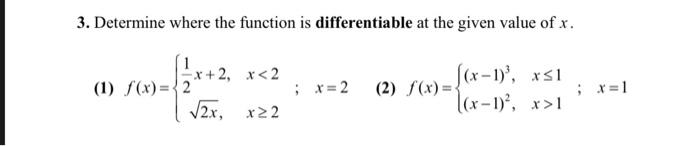### Create an Account

Home / Questions / 3. Determine where the function is differentiable at the given value of x. -2, x 1

# 3. Determine where the function is differentiable at the given value of x. -2, x 1

3. Determine where the function is differentiable at the given value of x. -2, x 1Apr 29 2021 View more View LessSubscribe To Get Solution